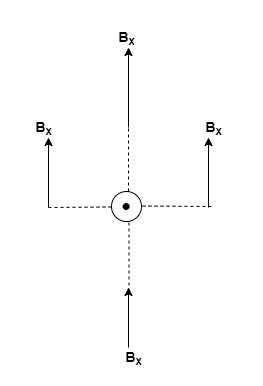# Problem: The position relative to the wires of the magnetic field of the current perpendicular to the Earth's magnetic field is east of the wire. Draw two vector diagrams for the magnetic field(s) felt by this compass: one with the current OFF and one with the current ON. Each diagram should show individual magnetic fields from any relevant sources, as well as a vector indicating the total magnetic field. For this problem, assume the current flows upward (in your drawing, this is out of the page).How would the compass in this scenario respond when you turn on the current? Explain your answer.

###### FREE Expert Solution

In this problem, we're going to let Bx be the horizontal component of the earth's magnetic field.

We're also going to let B be the magnetic field due to the current-carrying conductor.

When the current is OFF, we have:90% (293 ratings)###### Problem Details

The position relative to the wires of the magnetic field of the current perpendicular to the Earth's magnetic field is east of the wire. Draw two vector diagrams for the magnetic field(s) felt by this compass: one with the current OFF and one with the current ON. Each diagram should show individual magnetic fields from any relevant sources, as well as a vector indicating the total magnetic field. For this problem, assume the current flows upward (in your drawing, this is out of the page).

How would the compass in this scenario respond when you turn on the current? Explain your answer.